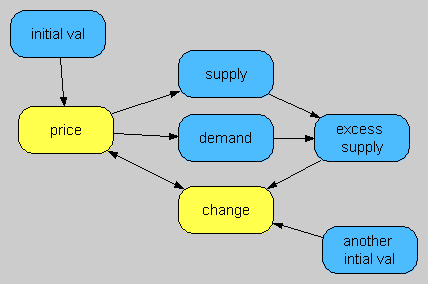# Error Messages/40336

## Example Error Text

A disallowed cyclic dependency involving the Iterate function in Price detected. You may have two nodes defined with Iterate functions, with each depending on the other. If you use two Iterate functions, one needs to be clearly nested inside the other. If you intended to have a single iteration involving two or more nodes, the Iterate function should appear only once.

## Cause

Here is an example influence diagram, where the yellow nodes are defined using the Iterate function:Here each Iterate depends on the other. Analytica doesn't allow that because it creates several problems. If you start by evaluating price, the first iteration begins, requesting change at iteration i. Change then starts its iteration, and requests price at its iteration i, which isn't yet fully computed. At that point, there would be two potential ways to proceed. One option would be to start the iteration for Price again from the start. That, of course, would form an infinite recursion that would never terminate. The other option would be to use the "current" value from Price's previous iteration. While that approach would eventually terminate and yield a result, it is flawed because now the answer depends on the order of evaluation. Had we started by evaluating change first, we'd end up with different values. This violates the basic premise that is well-entrenched in Analytica that the definition of a variable determines its result. Said another way, the definition is an "implicit" description of the "explicit" value of a variable. So, the bottom line is that this mutual-dependency of Iterate loops is disallowed.

In contrast, you can have an Iterate nested within an Iterate. If A is nested within B, then A is a descendant (a dependent of) B, but B is not a descendant of A.

## Remedies

You may have been thinking that both price and change need to be computed by the same iteration, so you wrapped both in an iterate. Both may have "initial" guesses and both may have an update formula. That is fine, but you need to do this with a single Iterate. One variable needs to be the "master" for the iteration. Sometimes I even separate this "master" as its own variable, with a name like "iteration", and have it update all variables.

One trick that works particularly nicely when you have multiple variables that need to be updated in each iteration is to define all those with the ComputedBy function. Each is declared as ComputedBy(My_iteration). Then My_iteration is defined with the Iterate function, and uses assignment both in the initial condition and in the update formula to update all these variables:

Variable A := ComputedBy(My_iteration)
Variable B := ComputedBy(My_iteration)
Variable My_iteration :=
Iterate(A := A0; B := B0,
A := Next_A; B := Next_B,
A - Next_A < 1e-3 and B - Next_B < 1e-3,
1000)